TitleCollege Algebra
Tutorial 23B: Rational InequalitiesLearning Objectives

 After completing this tutorial, you should be able to: Solve rational inequalities using a sign graph of factors. Solve rational inequalities using the test-point method.Introduction

 In this tutorial we will be looking at solving rational inequalities using two different methods.  The methods of solving rational inequalities are very similar to solving quadratic inequalities.  If you need a review on solving quadratic inequalities, feel free to go to Tutorial 23A: Quadratic Inequalities.  And yes, we will be dealing with fractions (yuck!) as we go through the rational inequalities.  I think we are ready to start.Tutorial

 Rational Inequalities A rational inequality is one that can be written in one of the following standard forms: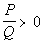or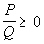or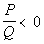or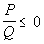Q does not equal 0.

 In other words, a rational inequality is in standard form when the inequality is set to 0.

 Solving Rational Inequalities Using a Sign Graph of the Factors

 This method of solving rational inequalities only works if the numerator and denominator factor.  If at least one of them doesn't factor then you will need to use the test-point method shown later on this page. This method works in the same fashion as it does with quadratic inequalities. If you need a review on solving quadratic inequalities, feel free to go to Tutorial 23A: Quadratic Inequalities. Be careful, it is really tempting to multiply both sides of the inequality by the denominator like you do when solving rational equations.  The problem is the expression in the denominator will have a variable, so we won't know what the denominator is equal to.  Remember that if we multiply both sides of an inequality by a positive number, it does not change the inequality.  BUT if we multiply both sides by a negative, it does change the sign of the inequality.  Since we don't know what sign we are dealing with we need to go about it the way described below.

 Step 1: Write the rational inequality in standard form.

 It is VERY important that one side of the inequality is 0. 0 is our magic number.  It is the only number that separates the negatives from the positives.  If an expression is greater than 0, then there is no doubt that its sign is positive.  Likewise, if it is less than 0, its sign is negative.  You can not say this about any other number.  Since we are working with inequalities, this idea will come in handy.  With this technique we will be looking at the sign of a number to determine if it is a solution or not.

 Step 2: Factor the numerator and denominator and find the values of x that make these factors equal to 0 to find the boundary points.

 The boundary point(s) will mark off where the rational expression is equal to 0.  This is like the cross over point.  0 is neither positive or negative. As mentioned above, this method of solving rational inequalities only works if the numerator and denominator factor.  If at least one of them doesn't factor then you will need to use the test-point method shown later on this page.

 Step 3: Use the boundary point(s) found in step 2 to mark off test intervals on the number line.

 The boundary point(s) on the number will create test intervals.

 Step 4: Find the sign of every factor in every interval.

 You can choose ANY value in an interval to plug into each factor.  Whatever the sign of the factor is with that value gives you the sign you need for that factor in that interval.  Make sure that you find the sign of every factor in every interval. Since the inequality will be set to 0,  we are not interested in the actual value that we get when we plug in our test points, but what SIGN (positive or negative) that we get.

 Step 5: Using the signs found in Step 4, determine the sign of the overall rational function in each interval.

Since the inequality will be set to 0,  we are not interested in the actual value that we get when we plug in our test points, but what SIGN (positive or negative) that we get.

When you look at the signs of your factors in each interval, keep in mind that they represent a product and/or quotient of the factors that make up your overall rational function.

You determine the sign of the overall rational function by using basic multiplication sign rules:

 The product or quotient of two factors that have the same sign is positive. The product or quotient of two factors that have the opposite signs is negative. This can be extended if you have more than two factors involved.

If the rational expression is less than or less than or equal to 0, then we are interested in values that cause the rational expression to be negative.

If the rational expression is greater than or greater than or equal to 0, then we are interested in values that cause our rational expression to be positive.

 Step 6: Write the solution set and graph.

 If you need a review on writing interval notation or graphing an inequality, feel free to go to Tutorial 22: Linear Inequalities.Example 1: Solve, write your answer in interval notation and graph the solution set: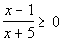.

 Step 1: Write the rational inequality in standard form.

 This rational inequality is already in standard form.

 Step 2: Factor the numerator and denominator and find the values of x that make these factors equal to 0 to find the boundary points.

 Numerator: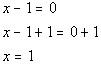*Set numerator = 0 and solve

 Denominator: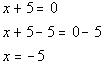*Set denominator = 0 and solve

 -5 and 1 are boundary points.

 Below is a graph that marks off the boundary points -5 and 1 and shows the three sections that those points have created on the graph.  Note that there is a open hole at -5.  Since that is the value that causes the denominator to be 0, we cannot include where x = -5.  Since our inequality includes where it is equal to 0, and 1 causes only the numerator to be 0 there is a closed hole at 1.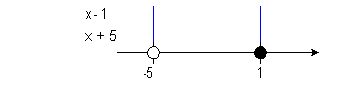Note that the two boundary points create three sections on the graph: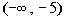,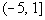, and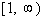.

 You can choose ANY point in an interval to represent that interval.  Remember that we are not interested in the actual value that we get, but what SIGN (positive or negative) that we get. If we chose a number in the first interval,, like -6 (I could have used -10, -25, or -10000 as long as it is in the interval), it would make both factors negative:   -6 - 1 = -7 and -6 + 5 = -1 If we chose a number in the second interval,, like 0 (I could have used -4, -1, or 1/2 as long as it is in the interval), it would make x - 1 negative and x + 5 positive:  0 - 1 = -1 and 0 + 5 = 5 If we chose a number in the third interval,, like 2 (I could have used 10, 25, or 10000 as long as it is in the interval), it would make both factors positive:   2 - 1 = 1 and 2 + 5 = 7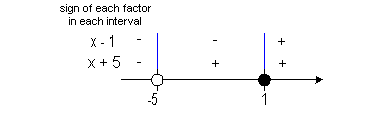Step 5: Using the signs found in Step 4, determine the sign of the overall rational function in each interval.

 In the first interval,, we have a negative divided by a negative, so the sign of the quadratic in that interval is positive. In the second interval,, we have a positive divided by a negative, so the sign of the quadratic in that interval is negative. In the third interval,, we have two positives, so the sign of the quadratic in that interval is positive. Keep in mind that our inequality is.  Since we are looking for the quadratic expression to be GREATER THAN OR EQUAL TO 0, that means we need our sign to be POSITIVE (OR O).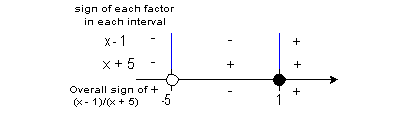Interval notation: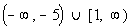Graph: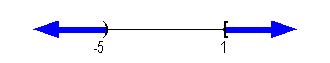*An open interval indicating all values less than -5  and a closed interval indicating all values greater then or equal to 1 *Visual showing all numbers less than -5 or greater then or equal to 1

 Solving Rational Inequalities Using the Test-Point Method

 The test-point method for solving rational inequalities works for any rational function that has a real number solution, whether the numerator or denominator factors or not. This method works in the same fashion as it does with quadratic inequalities. If you need a review on solving quadratic inequalities, feel free to go to Tutorial 23A: Quadratic Inequalities. Be careful, it is really tempting to multiply both sides of the inequality by the denominator like you do when solving rational equations.  The problem is the expression in the denominator will have a variable, so we won't know what the denominator is equal to.  Remember that if we multiply both sides of an inequality by a positive number, it does not change the inequality.  BUT if we multiply both sides by a negative, it does change the sign of the inequality.  Since we don't know what sign we are dealing with we need to go about it the way described below.

 Step 1: Write the rational inequality in standard form.

 It is VERY important that one side of the inequality is 0. 0 is our magic number.  It is the only number that separates the negatives from the positives.  If an expression is greater than 0, then there is no doubt that its sign is positive.  Likewise, if it is less than 0, its sign is negative.  You can not say this about any other number.  Since we are working with inequalities, this idea will come in handy.  With this technique we will be looking at the sign of a number to determine if it is a solution or not.

 Step 2:  Find the values of x that make the numerator and denominator equal to 0 to find the boundary points.

 The boundary point(s) will mark off where the rational expression is equal to 0.  This is like the cross over point.  0 is neither positive or negative.

 Step 3: Use the boundary point(s) found in Step 2 to mark off test intervals on the number line.

 The boundary point(s) on the number will create test intervals.

 Step 4: Test a point in each test interval found in Step 3 to see which interval(s) is part of the solution set.

 You can choose ANY point in an interval to represent it.  You need to make sure that you test one point from each interval.  Sometimes more than one interval can be part of the solution set. Since the inequality will be set to 0,  we are not interested in the actual value that we get when we plug in our test points, but what SIGN (positive or negative) that we get.  If the rational expression is less than or less than or equal to 0, then we are interested in values that cause the rational expression to be negative. If the rational expression is greater than or greater than or equal to 0, then we are interested in values that cause our rational expression to be positive.

 Step 5: Write the solution set and graph.

 If you need a review on writing interval notation or graphing an inequality, feel free to go to Tutorial 22: Linear Inequalities.Example 2: Solve, write your answer in interval notation and graph the solution set: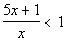.

 Step 1: Write the rational inequality in standard form.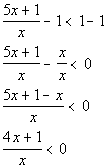*Inv. of add. 1 is sub. 1

 Step 2: Factor the numerator and denominator and find the values of x that make these factors equal to 0 to find the boundary points.

 Numerator: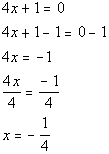*Set numerator = 0 and solve

 Denominator: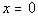*Set denominator = 0 and solve

 -1/4 and 0 are boundary points.

 Below is a graph that marks off the boundary points -1/4 and 0 and shows the three sections that those points have created on the graph.  Note that open holes were used on those two points since our original inequality did not include where it is equal to 0 and -1/4 makes the denominator 0.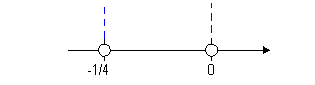Note that the two boundary points create three sections on the graph: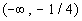,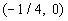, and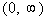.

 Step 4: Test a point in each test interval found in step 3 to see which interval(s) is part of the solution set.

 You can choose ANY point in an interval to represent that interval.  Remember that we are not interested in the actual value that we get, but what SIGN (positive or negative) that we get. Keep in mind that our original problem is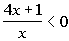.  Since we are looking for the quadratic expression to be LESS THAN 0, that means we need our sign to be NEGATIVE. From the interval, I choose to use -1 to test this interval: (I could have used -10, -25, or -10000 as long as it is in the interval)*Chose -1 from 1st interval to plug in for x

 Since 3 is positive and we are looking for values that cause our quadratic expression to be less than 0 (negative),would not be part of the solution.

 From the interval, I choose to use -1/5 to test this interval. (I could have used -1/6, -1/7, or -1/8 as long as it is in the interval)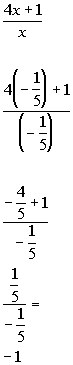*Chose -1/5 from 2nd interval to plug in for x

 Since -1 is negative and we are looking for values that cause our expression to be less than 0 (negative),would be part of the solution.

 From the interval, I choose to use 1 to test this interval. (I could have used 10, 25, or 10000 as long as it is in the interval)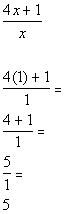*Chose 1 from 3rd interval to plug in for x

 Since 5 is positive and we are looking for values that cause our quadratic expression to be less than 0 (negative),would not be part of the solution.

 Interval notation:Graph: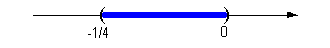*Open interval indicating all values between -1/4 and 0 *Visual showing all numbers between -1/4 and 0 on the number linePractice Problems

 These are practice problems to help bring you to the next level.  It will allow you to check and see if you have an understanding of these types of problems. Math works just like anything else, if you want to get good at it, then you need to practice it.  Even the best athletes and musicians had help along the way and lots of practice, practice, practice, to get good at their sport or instrument.  In fact there is no such thing as too much practice. To get the most out of these, you should work the problem out on your own and then check your answer by clicking on the link for the answer/discussion for that  problem.  At the link you will find the answer as well as any steps that went into finding that answer.Practice Problems 1a - 1b: Solve (using any method), write your answer in interval notation and graph the solution set.

 1a.(answer/discussion to 1a) 1b.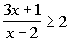(answer/discussion to 1b)Need Extra Help on these Topics?

 No appropriate web pages could be found to help you with the topics on this page.     Go to Get Help Outside the Classroom found in Tutorial 1: How to Succeed in a Math Class for some more suggestions.

Videos at this site were created and produced by Kim Seward and Virginia Williams Trice.
Last revised on Jan. 2, 2010 by Kim Seward.